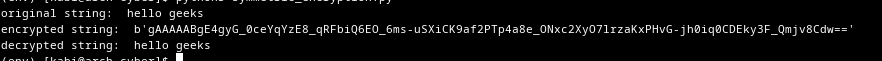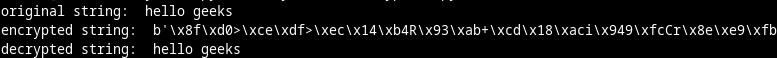Open in App
Not now

# How to Encrypt and Decrypt Strings in Python?

• Difficulty Level : Medium
• Last Updated : 08 Jun, 2022

Encryption:

Encryption is the process of encoding the data. i.e converting plain text into ciphertext. This conversion is done with a key called an encryption key.

Decryption:

Decryption is the process of decoding the encoded data. Converting the ciphertext into plain text. This process requires a key that we used for encryption.

We require a key for encryption. There are two main types of keys used for encryption and decryption. They are Symmetric-key and Asymmetric-key.

Symmetric-key Encryption:

In symmetric-key encryption, the data is encoded and decoded with the same key. This is the easiest way of encryption, but also less secure. The receiver needs the key for decryption, so a safe way need for transferring keys. Anyone with the key can read the data in the middle.

Example:

Install the python cryptography library with the following command.

`pip install cryptography`

Steps:

• Import Fernet
• Then generate an encryption key, that can be used for encryption and decryption.
• Convert the string to a byte string, so that it can be encrypted.
• Instance the Fernet class with the encryption key.
• Then encrypt the string with the Fernet instance.
• Then it can be decrypted with Fernet class instance and it should be instanced with the same key used for encryption.

## Python3

 `from` `cryptography.fernet ``import` `Fernet`   `# we will be encrypting the below string.` `message ``=` `"hello geeks"`   `# generate a key for encryption and decryption` `# You can use fernet to generate ` `# the key or use random key generator` `# here I'm using fernet to generate key`   `key ``=` `Fernet.generate_key()`   `# Instance the Fernet class with the key`   `fernet ``=` `Fernet(key)`   `# then use the Fernet class instance ` `# to encrypt the string string must` `# be encoded to byte string before encryption` `encMessage ``=` `fernet.encrypt(message.encode())`   `print``(``"original string: "``, message)` `print``(``"encrypted string: "``, encMessage)`   `# decrypt the encrypted string with the ` `# Fernet instance of the key,` `# that was used for encrypting the string` `# encoded byte string is returned by decrypt method,` `# so decode it to string with decode methods` `decMessage ``=` `fernet.decrypt(encMessage).decode()`   `print``(``"decrypted string: "``, decMessage)`

Output:Asymmetric-key Encryption:

In Asymmetric-key Encryption, we use two keys a public key and a private key. The public key is used to encrypt the data and the private key is used to decrypt the data. By the name, the public key can be public (can be sent to anyone who needs to send data). No one has your private key, so no one in the middle can read your data.

Example:

Install the python rsa library with the following command.

`pip install rsa`

Steps:

• Import rsa library
• Generate public and private keys with rsa.newkeys() method.
• Encode the string to byte string.
• Then encrypt the byte string with the public key.
• Then the encrypted string can be decrypted with the private key.
• The public key can only be used for encryption and the private can only be used for decryption.

## Python3

 `import` `rsa`   `# generate public and private keys with ` `# rsa.newkeys method,this method accepts ` `# key length as its parameter` `# key length should be atleast 16` `publicKey, privateKey ``=` `rsa.newkeys(``512``)`   `# this is the string that we will be encrypting` `message ``=` `"hello geeks"`   `# rsa.encrypt method is used to encrypt ` `# string with public key string should be ` `# encode to byte string before encryption ` `# with encode method` `encMessage ``=` `rsa.encrypt(message.encode(), ` `                         ``publicKey)`   `print``(``"original string: "``, message)` `print``(``"encrypted string: "``, encMessage)`   `# the encrypted message can be decrypted ` `# with ras.decrypt method and private key` `# decrypt method returns encoded byte string,` `# use decode method to convert it to string` `# public key cannot be used for decryption` `decMessage ``=` `rsa.decrypt(encMessage, privateKey).decode()`   `print``(``"decrypted string: "``, decMessage)`

Output:My Personal Notes arrow_drop_up
Related Articles